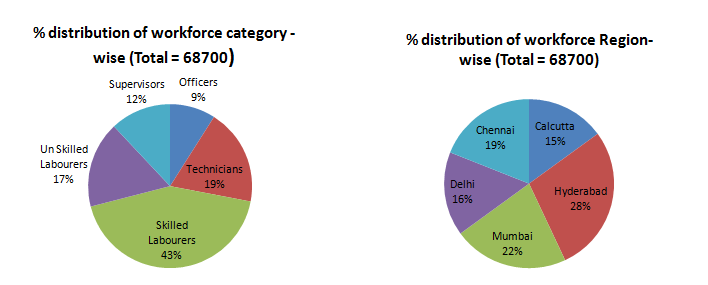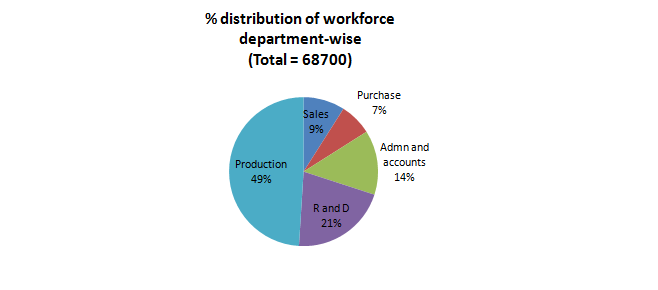Directions: Study the following pie-charts to answer these questions.Question 1: If 22% of the Production department persons are posted at Hyderabad region, then what % of Hyderabad workforce are in Production department?
(a) 38.5%
(b) 78%
(c) 68 %
(d) 22%

Question 2: How many supervisors are posted in Calcutta region?
(a) 1237
(b) 985
(c) 1144
(d) Data insufficient

Question 3: If 11% officers of the company are in Administration and Accounts department of which 75% are posted at Calcutta, then what percent of total officers of the company are posted at Administration and Accounts, Calcutta?
(a) 11.14%
(b) 8.25%
(c) 3.75%
(d) Data insufficient

Question 4: If under expansion programme, the company recruits 12% of workforce of Hyderabad and Mumbai regions, but 6% of workforce of Calcutta region retires, and workforce at other regions remains the same, then what will be the total workforce of the company?
(a) 65196
(b) 68238
(c) 72204
(d) 69430

Let the total Workforce = 100.

Workforce in Production = 49.

22% of Production = 22% of 49 = 10.78

So 10.78 workers in Hyderabad are in production department.

Total workforce in Hyderabad = 28

Therefore the percentage = (10.78/28) x 100 = 38.5%

The correct option is (a)

As percentage of supervisors posted in Calcutta region is not given so data is insufficient. The correct option is (d)

Total Officers in company is 68700 × 9/100 = 6183

The number of employees in the Administration and Accounts department = 11% of 6183 = 680.13

The number of employees in Administration and Accounts department posted in Calcutta = 75% of 680.13 = 510

So the required percentage = (510/6183) × 100 = 8.25%

The correct option is (b)

The total workforce at Hyderabad = 28% of 68700 = 19236

The total workforce at Mumbai = 22% of 68700 = 15114

The total workforce at Calcutta = 15% of 68700 = 10305

So the new recruitment = 12% of (19236 + 15114) = 12% of 34350 = 4122

The total retirement = 6% of 10305 = 618.3

Hence the total workforce = 68700 + 4122 – 618.3 = 72203.7 = 72204

The correct option is (c)

### Want to explore more Data Interpretation Sets?

Get Posts Like This Sent to your Email
Updates for Free Live sessions and offers are sent on mail. Don't worry: we do not send too many emails..:)
Get Posts Like This Sent to your Email
Updates for Free Live sessions and offers are sent on mail. Don't worry: we do not send too many emails..:)